## Sum( and Seq( commands on your calculator

Can you find the sum of the following series?$\displaystyle \sum_{i=1}^{15}\dfrac{2n+1}{3n-2}$

This is neither an arithmetic nor a geometric series, so you don’t have a formula for it. This would be a tedious problem to do by hand. Fortunately, your graphing calculator can do these problems quickly and efficiently.

There are two functions you need to use on your calculator. The seq( command creates a sequence of terms based on a rule that you give. The sum( command adds together the terms in a sequence. Both functions are found on the LIST menu on your calculator. The seq( command is on the OPS submenu and the sum( command is on the MATH submenu.

To sum a series, you combine the two commands. If you have the new operating system on your calculator, it will prompt you for the entries when you select the seq( command. If you have the old operating system, you need to know the syntax for the command. The syntax for the series above is:

sum(seq((2x + 1)/(3x – 2),x,1,15))

Note that the seq( command has four parameters in the parentheses. From left to right, these are 1) the rule for the nth term of the sequence; 2) the variable name; 3) the first value of the variable; and 4) the final value of the variable. Now all you need to do is type this in to your calculator and let it do the crunching:

## The “parent” functions

Do you know your parent functions? I’m surprised every year when some of my students don’t know how to graph some of the elementary functions they’ve learned in class. Your chances for success in precalc and calculus are significantly better if you memorize the shapes of these ten functions. No excuses, no shortcuts. Just learn them.

y = xn (Power functions)

y = |x| (absolute value function)

y = ⌊x⌋ (Greatest integer function)

y = √x

y = 1/x

y= bx (exponential function – growth and decay)

y = log x

y = sin x and y = cos x

y = tan x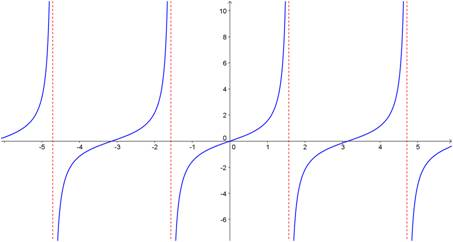## Calculating probabilities as a fraction

The first day you are asked to find probabilities, the questions are relatively simple:

• What is the probability of rolling a 2 or a 3 on a standard die? [Ans: 2/6 = 1/3]
• What is the probability of drawing a five from a standard deck of cards? [Ans: 4/52 = 1/13]

But very quickly, the problems get a lot more complicated:

• What is the probability of flipping a coin ten times and getting exactly 8 heads?
• What is the probability of being dealt a full house (three of a kind plus a pair) in a five card poker hand?
• A class has 14 boys and 12 girls. If the teacher randomly creates a new seating chart, what is the probability that the six students in the front row comprise exactly four boys and two girls?

How do you attack these problems? The best way is to think of probability as a fraction. The numerator counts all the ways the specified event can occur, and the denominator counts all the ways any event can occur:

Any event

Then you use combinatorics to count all the possibilities on the top and on the bottom. Let’s see how we can use this method to answer the three questions posed above:

(Note that this question could also be treated as a binomial probability and solved that way.)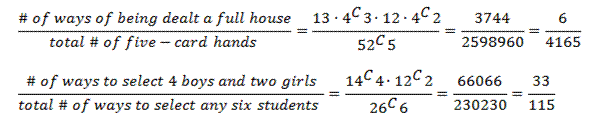## Factoring sums and differences of fifth powers (and higher!) when the power is odd

Occasionally, you are required to factor a polynomial that is in the form of a sum or difference of two power terms (i.e., x– yn). In my last post, I showed you a simple procedure you can learn to help you factor the binomial if the value of the power is 4 or greater and the power is even. [If you are reading this post, you should already be familiar with factoring the difference of two squares and the difference of two cubes. Check this website for posts on those procedures.] In this post, I show you a procedure (more complicated than the others, I admit) that you can use to factor these binomials when the power is odd.

First, let’s see how this works on some examples:

Do you see the pattern? When factoring a– bn or a+ bn (for n odd), there is always a linear term a ± b, where the plus or minus sign is the same as in the original binomial. The remaining factor is a little more complicated, but it does have a simple pattern. Each term consists of the first term in the binomial (with its exponent decreasing from n – 1 to 0) and the second term in the binomial with its exponent increasing from 0 to n – 1. In the first example above, the x term decreased from x4 to x0. The 2 term increased from 20 to 24. If you started with the difference of two powers, all of the signs in the second factor are plus signs. If you started with the sum of the two powers, the first sign in the second factor is a minus sign and the signs alternate after that.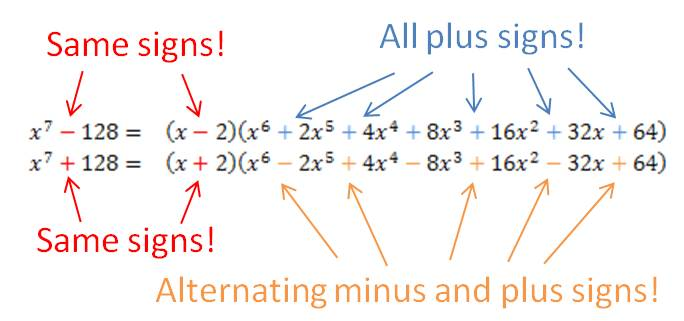## Factoring differences of fourth powers (and higher!) when the power is even

Occasionally, you are required to factor a polynomial that is in the form of a difference of two power terms (i.e., xn – yn). There is a simple procedure you can learn to help you factor the polynomial if the value of the power is 4 or greater and the power is even. [If you are reading this post, you should already be familiar with factoring the difference of two squares and the difference of two cubes. Check this website for posts on those procedures.]

First, let’s see how this works on some examples:

You can see that whenever the power is even, you treat the binomial as the difference of two squares and find its factors. If the factors are still the sum and difference of perfect squares, you repeat the process with the term that is the difference of two squares. If the factors are now the sum and difference of perfect cubes, you follow the method for factoring the sum or difference of two cubes. If the powers are sums and differences of odd powers higher than 3, there is another procedure to factor them. You can find another post on this website that shows that procedure.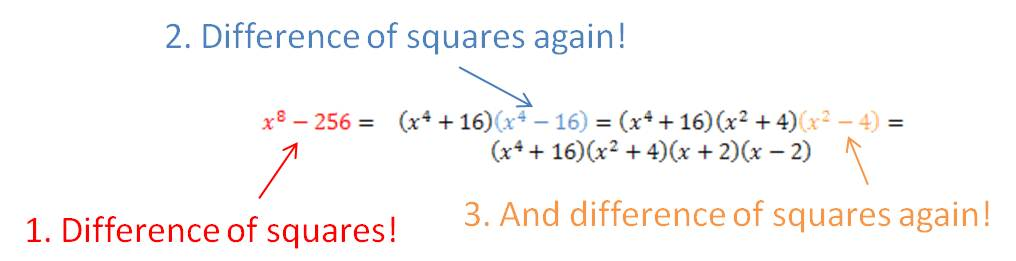## Translating functions

Any function f(x) is translated h units to the right when you replace x with x-h. Any function is translated k units up when you replace y with y-k. This is the basis of the vertex form of parabolas and standard form of the other conic sections, but it will help you graph almost any function you encounter without having to write out a “tree” first. If you know what the “parent” functions look like (and by the time you get to Algebra 2, you should memorize the parent functions), then you can graph translations without too much trouble.

Example 1: Graph

f(x) = ln (x-3).

Here the blue dotted graph is the parent function f(x) = ln (x). The red graph shows the solution, by translating the graph 3 units to the right.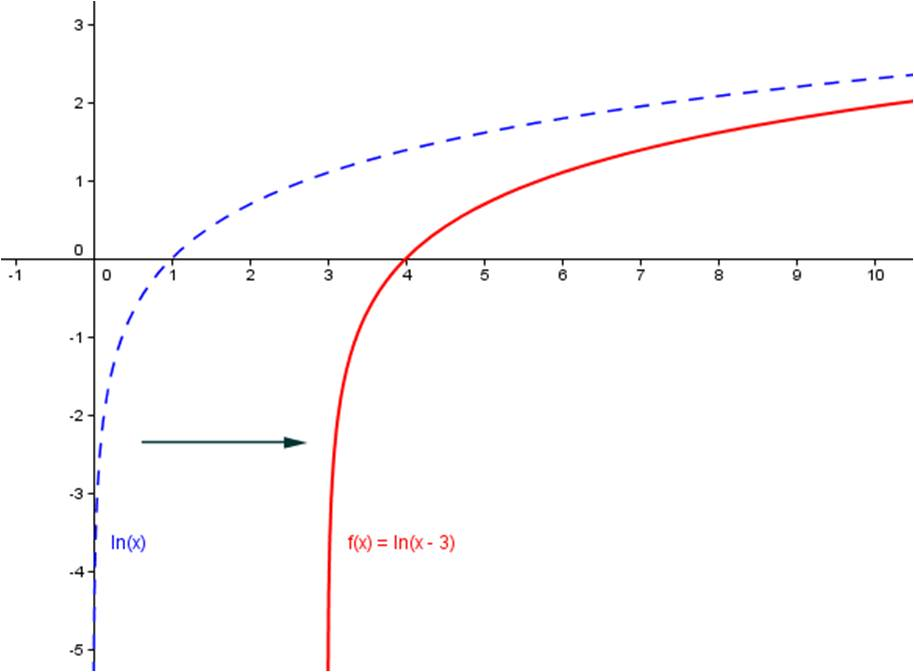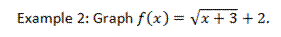In the graphs below, the parent function has been translated 3 units to the left and 2 units up.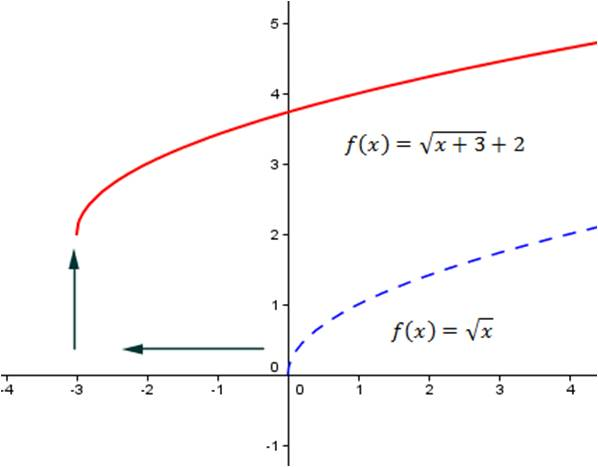## Factoring the sum or difference of two cubes

The sum or difference of two cubes can always be factored as follows:$(x^3+y^3)=(x+y)(x^2-xy+y^2)$$(x^3-y^3)=(x-y)(x^2+xy+y^2)$

These formulas are not too easy to figure out on your own, so your best approach is to memorize them. But how do you keep straight where the plus and minus signs go? Some students like to use the acronym “SOAP”, which stands for Same-Opposite-Always Positive. This means that the first sign is the same as the sign in the sum or difference of the cubes, the second sign is the opposite of this sign, and the third sign is always positive.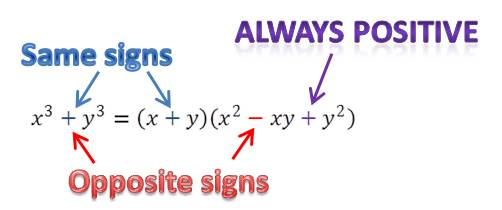Blue Taste Theme created by Jabox